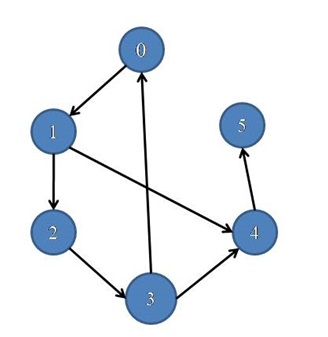# Cycle Detection in a Directed Graph

In this article, we are going to see how to find whether cycle exists or not in a directed graph?
Submitted by Souvik Saha, on March 25, 2019

What to Learn?

How to detect a cycle in a Directed graph?

In the following graph,It has a cycle 0-1-2-3-0

(1-2-3-4-1 is not cycle since edge direction is 1->4, not 4->1)

Algorithm:

Here we use a recursive method to detect a cycle in a graph.

1. We check presence of a cycle starting by each and every node at a time.
2. For each node
1. Whenever we visited one vertex we mark it.
2. Except the starting node, we go for all its adjacent nodes.
3. If there is any marked vertex present that means there is a cycle present in the graph.
```    Check(current node){
visit[curr_node]=true;
for( all the adjacent vertices ){
if(not visited yet) then
else if( the vertex is already visited) then
There is a cycle in the graph
}
}
```

C++ implementation:

```#include <bits/stdc++.h>
using namespace std;

void cycle_check(list<int>*,int);

//	Make a pair between vertex x and vertex y
ls[x].push_back(y);
return;
}

void check_cycle_util(list<int> *ls,bool *visit,int curr_node,int &temp){
visit[curr_node]=true;
list<int>::iterator it;
for(it=ls[curr_node].begin();it!=ls[curr_node].end();it++){
if(!visit[*it]){
check_cycle_util(ls,visit,*it,temp);
}
else{
if(temp==0){
temp=1;
cout<<"There is a cycle in the graph\n";
break;
}
}
}
}
//checking the cycles in a graph
void cycle_check(list<int>*ls,int num){
bool *visit=new bool[num];
int temp=0;
for(int i=0;i<num;i++)
visit[i]=false;
for(int i=0;i<num;i++){
if(!visit[i] && temp==0){
check_cycle_util(ls,visit,i,temp);
}
}
}

int main(){
int num;
cout<<"Enter the no. of vertices :";
cin>>num;
list<int> *ls=new list<int>[num];
cycle_check(ls,6);

return 0;
}
```

Output

```Enter the no. of vertices : 6
There is a cycle in the graph
```